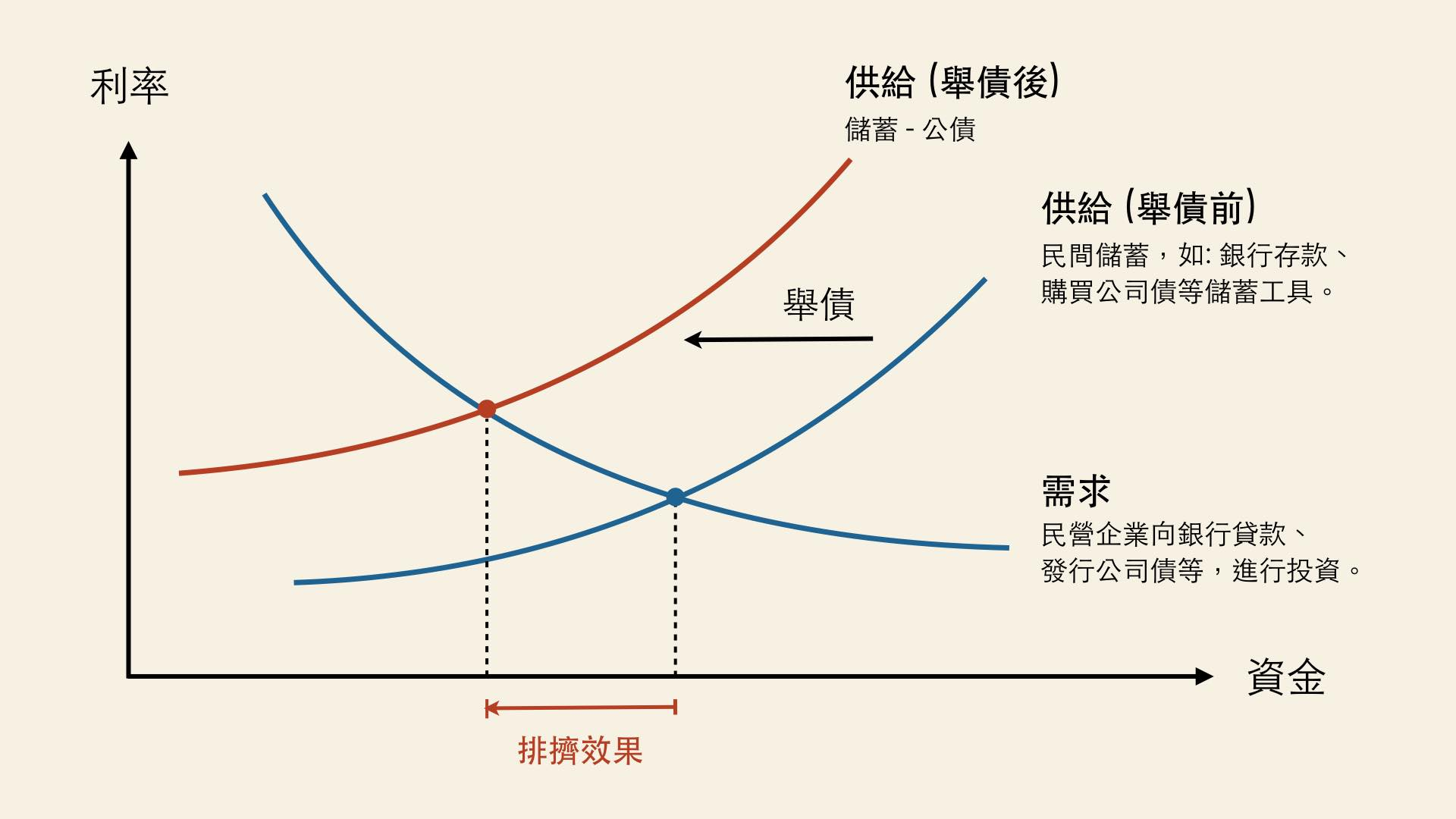## 推動「前瞻」的理由？

1. 台灣近年公共投資 (包含政府投資及公營事業投資) 呈負成長，所以應該要擴大公共投資，以帶動民間投資與創新。
2. 根據世界經濟論壇 (World Economic Forum) 的「2016-2017 年全球競爭力報告」，台灣在「基礎建設」這個項目的分數比新加坡、香港、南韓低，所以要針對「基礎建設」擴大政府投資。

## 公共投資的「乘數效果」?

$1000 + 1000 \times 0.4 + 1000 \times 0.4^2 + 1000 \times 0.4^3+ …$

$= 1000/(1-0.4) = 1670 億元$

## 公共投資的「排擠效果」## 結語

1. 實證研究仍無法確知乘數效果大小，考慮「消費平滑」理論後，公共投資的乘數效果甚至可能小於 1。
2. 即使乘數效果大於 1，政府的錢並非無中生有，就算舉債支應，將來仍需加稅，屆時仍會有「反向的乘數效果」，因此長期而言，乘數效果對總體經濟並無助益。
3. 舉債支應公共投資會扭曲私部門的「資金市場」，間接對私部門投資產生「排擠效果」，公共投資不一定能帶動民間投資。

1. 怎麼算出來的？如果整體經濟的總收入增加了 $$x$$ 元，那麼: $x = 1000 + 1000 \times 0.4 + 1000 \times 0.4^2 + 1000 \times 0.4^3 + …$ 等號的左右同乘以 0.4，得到: $0.4x = 1000 \times 0.4 + 1000 \times 0.4^2 + 1000 \times 0.4^3 + 1000 \times 0.4^4 + …$ $x-0.4x = 1000$ $x = 1000/(1-0.4)$ ↩︎